/

### Function Description

Returns an array ofelements containing the quantiles predicted by the 4th moment Cornish-Fisher asymptotic expansion for a given input array of probability levels (withelements), for a given mean, standard deviation, skew and (excess) kurtosis.

The Cornish-Fisher asymptotic expansion is a methodology for predicting the shape of a (univariate) distributional form merely from the moments of the distribution. The 4th moment variant uses the mean, standard deviation, skew and (excess) kurtosis of the distribution.

For standardised returns (zero mean, unit standard deviation), the 4th moment variant involves estimating the shape of the quantile-quantile plot of the actual versus (standard) Normal distribution using the following cubic equation, where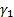is the skew and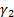is the (excess) kurtosis of the distribution: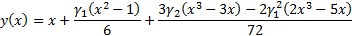In the more generalised case where the mean,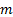, is not necessarily zero and the standard deviation,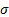is not necessarily unity the 4th moment variant involves estimating the shape of the quantile-quantile plot as follows: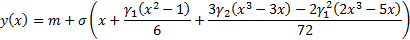Contents | Prev | Next